• 傅里叶变换与拉普拉斯变换的关系：

傅里叶变换与拉普拉斯变换的关系：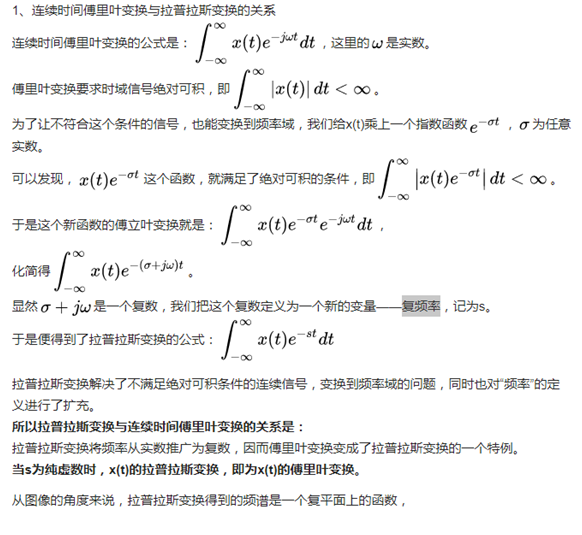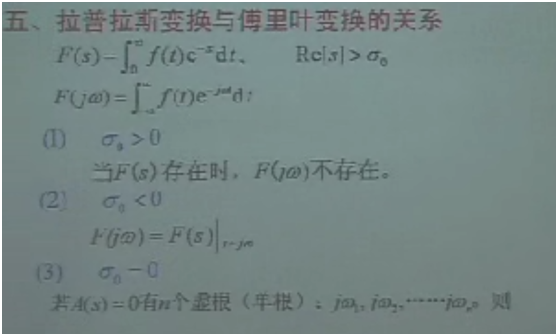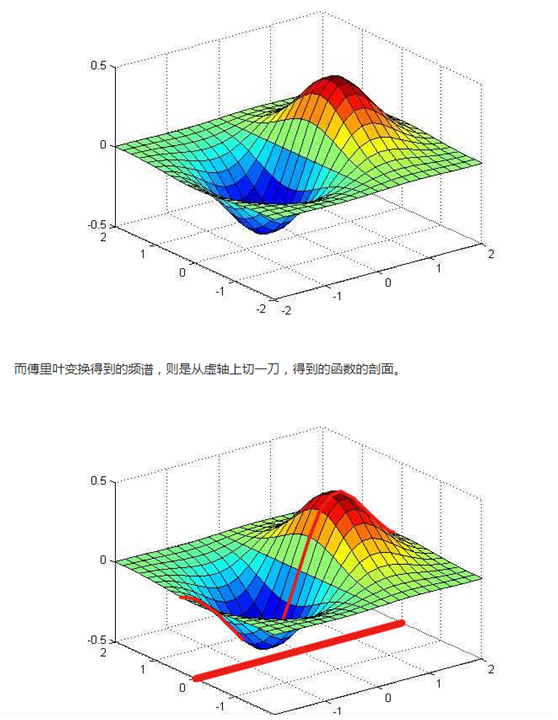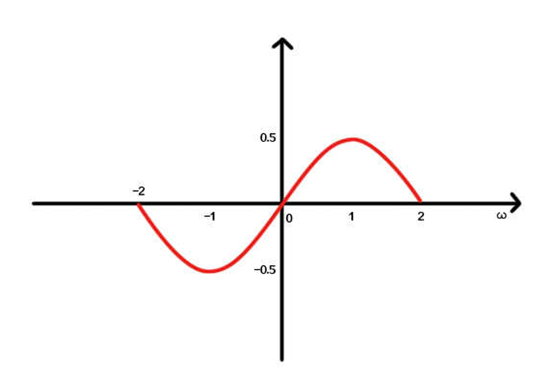展开全文• 傅里叶变换、拉普拉斯变换、Z变换公式和性质表格汇总。傅里叶变换、拉普拉斯变换、Z变换公式和性质表格汇总。傅里叶变换、拉普拉斯变换、Z变换公式和性质表格汇总。傅里叶变换、拉普拉斯变换、Z变换公式和性质表格...
• 傅里叶变换与拉普拉斯变换的概念理解
傅里叶变换与拉普拉斯变换的概念理解
傅里叶变换在物理学、数论、组合数学、信号处理、概率论、统计学、密码学、声学、光学、海洋学、结构动力学等领域都有着广泛的应用（例如在信号处理中，傅里叶变换的典型用途是将信号分解成幅值分量和频率分量）。      傅里叶变换能将满足一定条件的某个函数表示成三角函数（正弦和/或余弦函数）或者它们的积分的线性组合。在不同的研究领域，傅里叶变换具有多种不同的变体形式，如连续傅里叶变换和离散傅里叶变换。
傅里叶变换是一种解决问题的方法，一种工具，一种看待问题的角度。理解的关键是：一个连续的信号可以看作是一个个小信号的叠加，从时域叠加与从频域叠加都可以组成原来的信号，将信号这么分解后有助于处理。      我们原来对一个信号其实是从时间的角度去理解的，不知不觉中，其实是按照时间把信号进行分割，每一部分只是一个时间点对应一个信号值，一个信号是一组这样的分量的叠加。傅里叶变换后，其实还是个叠加问题，只不过是从频率的角度去叠加，只不过每个小信号是一个时间域上覆盖整个区间的信号，但他确有固定的周期，或者说，给了一个周期，我们就能画出一个整个区间上的分信号，那么给定一组周期值（或频率值），我们就可以画出其对应的曲线，就像给出时域上每一点的信号值一样，不过如果信号是周期的话 ，频域的更简单，只需要几个甚至一个就可以了，时域则需要整个时间轴上每一点都映射出一个函数值。      傅里叶变换就是将一个信号的时域表示形式映射到一个频域表示形式；逆傅里叶变换恰好相反。这都是一个信号的不同表示形式。它的公式会用就可以，当然把证明看懂了更好。
对一个信号做傅里叶变换，可以得到其频域特性，包括幅度和相位两个方面。幅度是表示这个频率分量的大小，那么相位呢，它有什么物理意义？频域的相位与时域的相位有关系吗？信号前一段的相位（频域）与后一段的相位的变化是否与信号的频率成正比关系。      傅里叶变换就是把一个信号，分解成无数的正弦波（或者余弦波）信号。也就是说，用无数的正弦波，可以合成任何你所需要的信号。      想一想这个问题：给你很多正弦信号，你怎样才能合成你需要的信号呢？答案是要两个条件，一个是每个正弦波的幅度，另一个就是每个正弦波之间的相位差。所以现在应该明白了吧，频域上的相位，就是每个正弦波之间的相位。        傅里叶变换用于信号的频率域分析，一般我们把电信号描述成时间域的数学模型，而数字信号处理对信号的频率特性更感兴趣，而通过傅立叶变换很容易得到信号的频率域特性。      傅里叶变换简单通俗理解就是把看似杂乱无章的信号考虑成由一定振幅、相位、频率的基本正弦（余弦）信号组合而成，傅里叶变换的目的就是找出这些基本正弦（余弦）信号中振幅较大（能量较高）信号对应的频率，从而找出杂乱无章的信号中的主要振动频率特点。如减速机故障时，通过傅里叶变换做频谱分析，根据各级齿轮转速、齿数与杂音频谱中振幅大的对比，可以快速判断哪级齿轮损伤。
拉普拉斯变换，是工程数学中常用的一种积分变换。      它是为简化计算而建立的实变量函数和复变量函数间的一种函数变换。对一个实变量函数作拉普拉斯变换，并在复数域中作各种运算，再将运算结果作拉普拉斯反变换来求得实数域中的相应结果，往往比直接在实数域中求出同样的结果在计算上容易得多。拉普拉斯变换的这种运算步骤对于求解线性微分方程尤为有效，它可把微分方程化为容易求解的代数方程来处理，从而使计算简化。在经典控制理论中，对控制系统的分析和综合，都是建立在拉普拉斯变换的基础上的。
引入拉普拉斯变换的一个主要优点，是可采用传递函数代替微分方程来描述系统的特性。这就为采用直观和简便的图解方法来确定控制系统的整个特性（见信号流程图、动态结构图）、分析控制系统的运动过程（见奈奎斯特稳定判据、根轨迹法），以及综合控制系统的校正装置（见控制系统校正方法）提供了可能性。      拉普拉斯变换在工程学上的应用：应用拉普拉斯变换解常变量齐次微分方程，可以将微分方程化为代数方程，使问题得以解决。在工程学上，拉普拉斯变换的重大意义在于：将一个信号从时域上，转换为复频域（s域）上来表示；在线性系统，控制自动化上都有广泛的应用。      在数字信号处理中，Z变换是一种非常重要的分析工具。但在通常的应用中，我们往往只需要分析信号或系统的频率响应，也即是说通常只需要进行傅里叶变换即可。那么，为什么还要引进Z变换呢？
在数字信号处理中，Z变换是一种非常重要的分析工具。但在通常的应用中，我们往往只需要分析信号或系统的频率响应，也即是说通常只需要进行傅里叶变换即可。那么，为什么还要引进Z变换呢？
Z变换和傅里叶变换之间有存在什么样的关系呢？傅里叶变换的物理意义非常清晰：将通常在时域表示的信号，分解为多个正弦信号的叠加。每个正弦信号用幅度、频率、相位就可以完全表征。傅里叶变换之后的信号通常称为频谱，频谱包括幅度谱和相位谱，分别表示幅度随频率的分布及相位随频率的分布。在自然界，频率是有明确的物理意义的，比如说声音信号，男同胞声音低沉雄浑，这主要是因为男声中低频分量更多；女同胞多高亢清脆，这主要是因为女声中高频分量更多。对一个信号来说，就包含的信息量来讲，时域信号及其相应的傅里叶变换之后的信号是完全一样的。那傅里叶变换有什么作用呢？因为有的信号主要在时域表现其特性，如电容充放电的过程；而有的信号则主要在频域表现其特性，如机械的振动，人类的语音等。若信号的特征主要在频域表示的话，则相应的时域信号看起来可能杂乱无章，但在频域则解读非常方便。在实际中，当我们采集到一段信号之后，在没有任何先验信息的情况下，直觉是试图在时域能发现一些特征，如果在时域无所发现的话，很自然地将信号转换到频域再看看能有什么特征。信号的时域描述与频域描述，就像一枚硬币的两面，看起来虽然有所不同，但实际上都是同一个东西。正因为如此，在通常的信号与系统的分析过程中，我们非常关心傅里叶变换。
既然人们只关心信号的频域表示，那么Z变换又是怎么回事呢？要说到Z变换，可能还要先追溯到拉普拉斯变换。拉普拉斯变换是以法国数学家拉普拉斯命名的一种变换方法，主要是针对连续信号的分析。拉普拉斯和傅里叶都是同时代的人，他们所处的时代在法国是处于拿破仑时代，国力鼎盛。在科学上也取代英国成为当时世界的中心，在当时众多的科学大师中，拉普拉斯、拉格朗日、傅里叶就是他们中间最为璀璨的三颗星。傅里叶关于信号可以分解为正弦信号叠加的论文，其评审人即包括拉普拉斯和拉格朗日。
回到正题，傅里叶变换虽然好用，而且物理意义明确，但有一个最大的问题是其存在的条件比较苛刻，比如时域内绝对可积的信号才可能存在傅里叶变换。拉普拉斯变换可以说是推广了这以概念。在自然界，指数信号exp(-x)是衰减最快的信号之一，对信号乘上指数信号之后，很容易满足绝对可积的条件。因此将原始信号乘上指数信号之后一般都能满足傅里叶变换的条件，这种变换就是拉普拉斯变换。这种变换能将微分方程转化为代数方程，在18世纪计算机还远未发明的时候，意义非常重大。从上面的分析可以看出，傅里叶变换可以看做是拉普拉斯的一种特殊形式，即所乘的指数信号为exp(0)。也即是说拉普拉斯变换是傅里叶变换的推广，是一种更普遍的表达形式。在进行信号与系统的分析过程中，可以先得到拉普拉斯变换这种更普遍的结果，然后再得到傅里叶变换这种特殊的结果。这种由普遍到特殊的解决办法，已经证明在连续信号与系统的分析中能够带来很大的方便。     Z变换可以说是针对离散信号和系统的拉普拉斯变换，由此我们就很容易理解Z变换的重要性，也很容易理解Z变换和傅里叶变换之间的关系。Z变换中的Z平面与拉普拉斯中的S平面存在映射的关系，z=exp(Ts)。在Z变换中，单位圆上的结果即对应离散时间傅里叶变换的结果。

展开全文• 本文是知乎上大佬的学习， 其知乎名为逸珺：傅里叶变换、拉普拉斯变换、Z 变换的联系是什么？为什么要进行这些变换？ - 知乎 三者关系如下 如下图所示，傅里叶变换是拉普拉斯变换的特殊形式，Z变换是拉普拉斯...
本文是对知乎上大佬的学习， 其知乎名为逸珺：傅里叶变换、拉普拉斯变换、Z 变换的联系是什么？为什么要进行这些变换？ - 知乎
三者关系如下
如下图所示，傅里叶变换是拉普拉斯变换的特殊形式，Z变换是拉普拉斯变换的离散形式。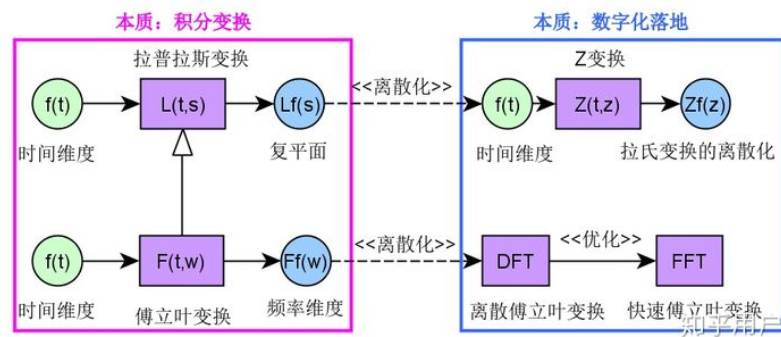要理解三种变换的联系区别，首先要理解什么是数学变换，什么是积分变换
因为：傅里叶变换和及拉普拉斯变换的本质都是基本变换

什么是数学变换

数学变换：指函数从原向量空间变换为另一个向量空间，或从集合x到另一个集合Y的可逆函数。
如旋转变换，平移，对称等等
数学中还有很多数学变换，其本质都可以看成是将函数f(x)利用变换因子进行的一种数学映射，其变换结果其函数的自变量有可能还是原来的几何空间，或许会变成其他的向量空间，比如傅立叶变换就从时域变换为频域。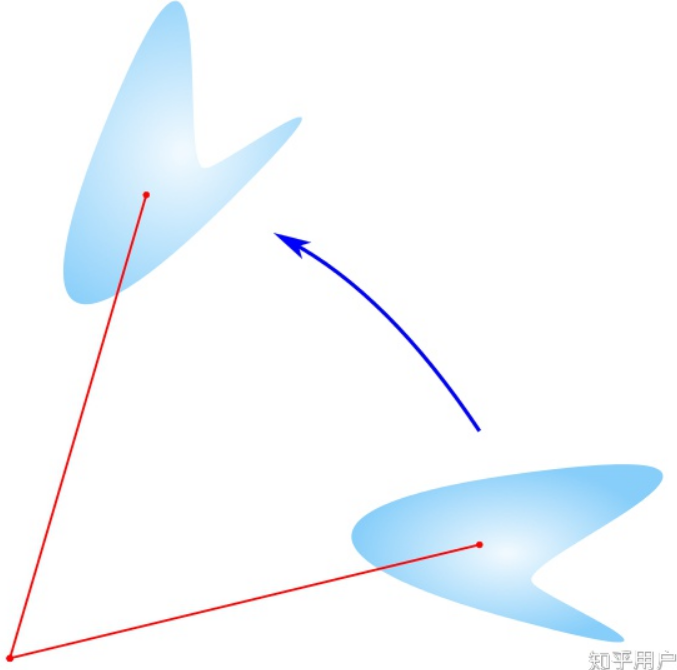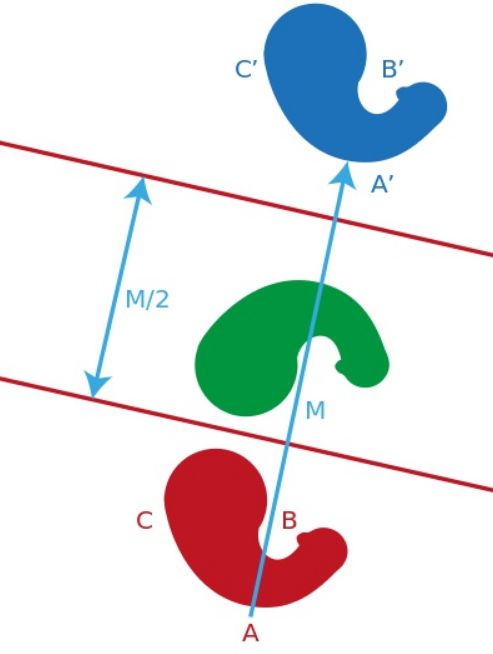而傅立叶变换与拉普拉斯变换本质上都是对连续函数的一种积分变换，那么什么是积分变换呢？
为何积分变换:
积分变换通过积分将一个函数从其原始函数空间映射到另一个函数空间，其中原始函数的某些属性可能比原始函数空间更容易表征和操作。通常可以通过逆变换变回原函数。
通常积分变换，假定对于函数为自变量t的函数f(t)，都类似具有以下的范式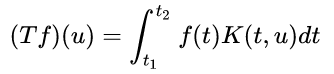函数f(t)是该变换的输入，(Tf)(u)为变换的输出，因此积分变换一般也称为一种特定的数学运算符。而函数K(t,u)称为积分核函数(kernel function)。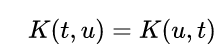变换可逆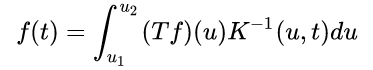观察正逆变换可知：
核函数刚好两个自变量交换位置正变换是对原函数f(t)在时间t维度上进行积分逆变换是在变换后的函数在u维度上进行积分
傅里叶级数
它可以将任何周期信号分解为一个或者多个正弦函数的集合。
其前为：周期信号,其周期为T。若f(t)在一个周期的能量是有限的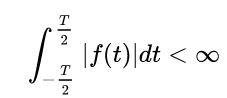可以将f(t)展开为傅立叶级数。计算如下：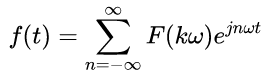F(kw)为傅里叶级数的系数，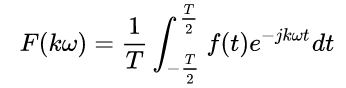或者利用欧拉公式，将原信号展开为正弦函数和的形式。
上式中的k表示第k次谐波。
什么是傅里叶变换
假设周期信号趋向于无穷大，则谱线间隔趋向于无穷小，周期信号变为非周期信号，谱线变成连续。前提是该函数绝对可积。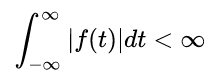傅里叶变换为：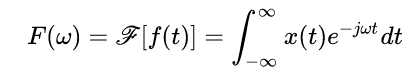其反变换为：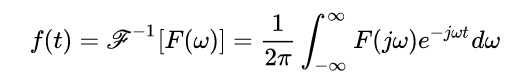为什么之前说傅里叶变换本质是积分变换呢，这里可以看到傅里叶变换的核函数为：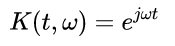核函数有两个自变量，t与Ω，一个表征时间，一个表征空间。
傅里叶变换与傅里叶级数的区别

傅立叶级数对应的是周期信号，而傅立叶变换则对应的是一个时间连续可积信号（不一定是周期信号）傅立叶级数要求信号在一个周期内能量有限，而后者则要求在整个区间能量有限傅立叶级数的对应是离散的，而傅立叶变换则对应Ω是连续的。
故：
F(jk)代表周期信号的第k次谐波的大小，F(jΩ)则是频谱密度概念。
拉普拉斯变换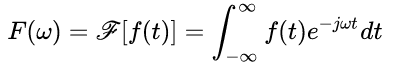那么如果对于函数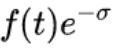其傅立叶变换为（这里描述单边拉普拉斯变换）：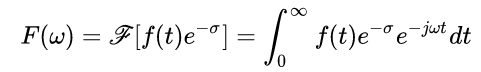上面公式整理如下：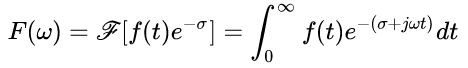令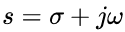,则上面的变换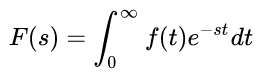核函数：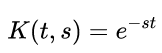傅里叶变换与拉普拉斯区别

拉普拉斯变换，将原函数从时间维度（不一定是时间维度，只是方便理解本文以常见的时间维度信号进行描述），映射为复平面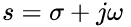傅立叶变换是拉普拉斯变换的特例，也即变换核函数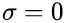时，拉普拉斯变换就变成傅立叶变换了。相当于只取虚部，实部为0.傅立叶变换是从原维度变换为频率维度，对于信号处理而言相当于将时域信号变换为频域进行分析，为信号处理提供了强大的数学理论基础及工具。拉普拉斯变换，将原维度变换为复频域，在电子电路分析以及控制理论中，为建立系统的数学描述提供了强大的数学理论基础，学过控制理论的一天到晚都与传递函数打交道，其本质就是拉普拉斯变换对系统的一种数学建模描述。为分析系统的稳定性、可控性提供了数学工具。

什么是Z变换
Z变换的本质是拉普拉斯变换的离散形式。对原信号进行抽样得到离散信号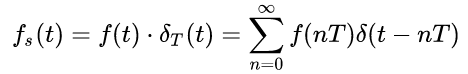然后进行拉普拉斯变换：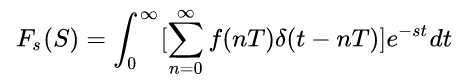利用冲激函数特性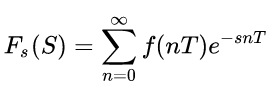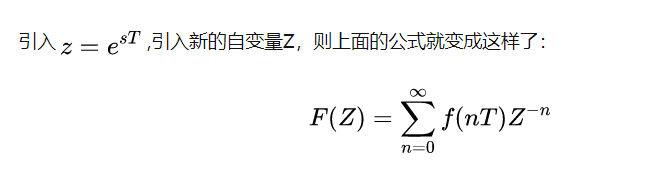Z变换的意义
利用Z变换很快能够将传递函数描述成差分方程形式，为计算机处理提供便利。


展开全文• 傅立叶变换以及拉普拉斯变换本质上都是积分变换，而傅立叶变换是拉普拉斯变换的特殊形式，而Z变换是拉普拉斯变换的离散形式。每种变换都有其应用价值，傅立叶变换在信号处理的频域分析中提供了强大的数学工具，而...

傅立叶变换以及拉普拉斯变换本质上都是积分变换，而傅立叶变换是拉普拉斯变换的特殊形式，Z变换则是拉普拉斯变换的离散形式。每种变换都有其应用价值，傅立叶变换在信号处理的频域分析中提供了强大的数学工具，而拉普拉斯变换在电子学、控制工程、航空航天等领域提供了建模、分析的数学分析工具；Z变换则将这些变换进而落地为数字实现提供数学理论依据。

1 欧拉公式
欧拉公式将三角函数与复指数函数巧妙地关联了起来，有下面的形式：

e

i

θ

=

cos

⁡

θ

+

i

sin

⁡

θ

e^{i\theta} =\cos \theta +i\sin \theta

其中，

e

e

为自然常数，

i

i

为虚数，

θ

\theta

则是以弧度为单位的参数（变量）。
下面给出一种常用的方式（级数）验证欧拉公式，在实数域里，有下面的麦克劳林级数：

e

x

=

1

+

x

+

1

2

!

x

2

+

1

3

!

x

3

+

⋯

sin

⁡

x

=

x

−

1

3

!

x

3

+

1

5

!

x

5

+

⋯

cos

⁡

x

=

1

−

1

2

!

x

2

+

1

4

!

x

4

+

⋯

把

x

=

i

θ

x = i\theta

带入

e

x

e^x

中有：

e

i

θ

=

1

+

i

θ

+

(

i

θ

)

2

2

!

+

(

i

θ

)

3

3

!

+

(

i

θ

)

4

4

!

+

(

i

θ

)

5

5

!

+

(

i

θ

)

6

6

!

+

(

i

θ

)

7

7

!

+

(

i

θ

)

8

8

!

+

⋯

=

1

+

i

θ

−

θ

2

2

!

−

i

θ

3

3

!

+

θ

4

4

!

+

i

θ

5

5

!

−

θ

6

6

!

−

i

θ

7

7

!

+

θ

8

8

!

+

⋯

=

(

1

−

θ

2

2

!

+

θ

4

4

!

−

θ

6

6

!

+

θ

8

8

!

−

⋯

)

+

i

(

θ

−

θ

3

3

!

+

θ

5

5

!

−

θ

7

7

!

+

⋯

)

=

cos

⁡

θ

+

i

sin

⁡

θ

\begin{aligned} e^{i\theta} & = 1 + i\theta + \frac{(i\theta)^2}{2!} + \frac{(i\theta)^3}{3!} + \frac{(i\theta)^4}{4!} + \frac{(i\theta)^5}{5!} + \frac{(i\theta)^6}{6!} + \frac{(i\theta)^7}{7!} + \frac{(i\theta)^8}{8!} + \cdots \\ & = 1 + i\theta - \frac{\theta^2}{2!} - \frac{i\theta^3}{3!} + \frac{\theta^4}{4!} + \frac{i\theta^5}{5!} - \frac{\theta^6}{6!} - \frac{i\theta^7}{7!} + \frac{\theta^8}{8!} + \cdots \\ & = \left( 1 - \frac{\theta^2}{2!} + \frac{\theta^4}{4!} - \frac{\theta^6}{6!} + \frac{\theta^8}{8!} - \cdots \right) + i\left(\theta-\frac{\theta^3}{3!} + \frac{\theta^5}{5!} - \frac{\theta^7}{7!} + \cdots \right) \\ &=\cos\theta + i\sin\theta \end{aligned}

当参数

x

x

等于

π

\pi

的时候，欧拉公式可简化为：

e

i

π

+

1

=

0

e^{i\pi}+1 =0

上式将5个微妙且看似无关的数学符号

e

、

i

、

π

、

0

、

1

e、i、\pi、0、1

紧密地联系了起来，其美妙之处让人称绝。
拓展：
自然底数

e

e

的介绍，请阅读：自然底数e怎么就“自然”了？虚数

i

i

的介绍，请阅读：虚数i真的很“虚”吗？

π

\pi

的介绍，请阅读：如何寻找到 π 的？欧拉公式的详细介绍，请阅读：欧拉公式，复数域的成人礼。

2 傅里叶变换
2.1 推导
（1）傅里叶级数         对于周期函数

f

(

x

)

f(x)

，根据傅里叶级数公式有：

f

(

x

)

=

a

0

+

∑

n

=

1

∞

(

a

n

cos

⁡

(

2

π

n

T

x

)

+

b

n

sin

⁡

(

2

π

n

T

x

)

)

,

a

0

∈

R

f(x)=a_{0}+\sum_{n=1}^{\infty}\left(a_{n} \cos \left(\frac{2 \pi n}{T} x\right)+b_{n} \sin \left(\frac{2 \pi n}{T} x\right)\right), a_{0} \in \mathbb{R}

通过欧拉公式，可以得到：

{

e

i

x

=

c

o

s

x

+

i

s

i

n

x

e

−

i

x

=

c

o

s

x

−

i

s

i

n

x

⟹

{

s

i

n

x

=

e

i

x

−

e

−

i

x

2

i

c

o

s

x

=

e

i

x

+

e

−

i

x

2

\begin{cases} e^{ix}= cosx+isinx \\ e^{-ix}= cosx-isinx \\ \end{cases} \implies \begin{cases} sinx = \frac{e^{ix} - e^{-ix}}{2i} \\ cosx = \frac{e^{ix} + e^{-ix}}{2} \\ \end{cases}

将上面的等式代入傅里叶级数公式，可以得到：

f

(

x

)

=

C

⋅

1

+

a

1

cos

⁡

(

2

π

T

x

)

+

b

1

sin

⁡

(

2

π

T

x

)

+

.

.

.

+

a

n

cos

⁡

(

2

π

n

T

x

)

+

b

n

sin

⁡

(

2

π

n

T

x

)

=

c

0

⋅

e

0

+

c

1

⋅

e

i

2

π

T

x

+

c

−

1

⋅

e

i

2

π

(

−

1

)

T

x

+

.

.

.

+

c

n

⋅

e

i

2

π

n

T

x

+

c

−

n

⋅

e

i

2

π

(

−

n

)

T

x

f(x)\;= C\cdot1+a_{1}\cos \left(\frac{2 \pi }{T} x\right)+b_{1}\sin \left(\frac{2 \pi }{T} x\right)+...+a_{n}\cos \left(\frac{2 \pi n}{T} x\right)+b_{n}\sin \left(\frac{2 \pi n}{T} x\right)\\ \quad \\ \qquad=c_{0}\cdot{e^0}+c_{1}\cdot{e^{i \frac{2\pi }{T}x}}+c_{-1}\cdot{e^{i \frac{2\pi (-1)}{T}x}}+...+c_{n}\cdot{e^{i \frac{2\pi n}{T}x}}+c_{-n}\cdot{e^{i \frac{2\pi (-n)}{T}x}}

从而可以得到：

f

(

x

)

=

∑

n

=

−

∞

∞

c

n

⋅

e

i

2

π

n

x

T

（

1

）

\displaystyle f(x)=\sum _{{n=-\infty }}^{\infty}c_{n}\cdot e^{{i{\tfrac {2\pi nx}{T}}}} \quad （1）

这里的

C

n

C_n

是一个复数，一般称为傅里叶系数，平时对频域的变换，一般改变的就是

C

n

C_n

，那么如何求

C

n

C_n

。
由公式（1）可以得到：

c

k

⋅

e

i

2

π

k

x

T

=

f

(

x

)

−

∑

n

=

−

∞

,

n

≠

k

∞

c

n

⋅

e

i

2

π

n

x

T

c_{k}\cdot e^{{i{\tfrac {2\pi kx}{T}}}} = f(x) -\sum_{{n=-\infty, n\not=k}}^{\infty}c_{n}\cdot e^{{i{\tfrac {2\pi nx}{T}}}}

两边同时乘以

e

−

i

2

π

k

x

T

e^{{-i{\tfrac {2\pi kx}{T}}}}

，可以得到：

c

k

=

f

(

x

)

⋅

e

−

i

2

π

k

x

T

−

∑

n

=

−

∞

,

n

≠

k

∞

c

n

⋅

e

i

2

π

(

n

−

k

)

x

T

c_{k} = f(x) \cdot e^{{-i{\tfrac {2\pi kx}{T}}}} -\sum_{{n=-\infty, n\not=k}}^{\infty}c_{n}\cdot e^{{i{\tfrac {2\pi (n-k)x}{T}}}}

两边积分可以得到：

∫

0

T

c

k

d

t

=

∫

0

T

[

f

(

x

)

⋅

e

−

i

2

π

k

x

T

−

∑

n

=

−

∞

,

n

≠

k

∞

c

n

⋅

e

i

2

π

(

n

−

k

)

x

T

]

d

t

T

c

k

=

∫

0

T

f

(

x

)

⋅

e

−

i

2

π

k

x

T

d

t

−

∑

n

=

−

∞

,

n

≠

k

∞

∫

0

T

c

n

⋅

e

i

2

π

(

n

−

k

)

x

T

d

t

\int_{0}^{T} c_{k}dt = \int_{0}^{T} [f(x) \cdot e^{{-i{\tfrac {2\pi kx}{T}}}} -\sum_{{n=-\infty, n\not=k}}^{\infty}c_{n}\cdot e^{{i{\tfrac {2\pi (n-k)x}{T}}}} ]dt \\ \quad Tc_{k} = \int_{0}^{T} f(x) \cdot e^{{-i{\tfrac {2\pi kx}{T}}}} dt - \sum_{{n=-\infty, n\not=k}}^{\infty} \int_{0}^{T} c_{n}\cdot e^{{i{\tfrac {2\pi (n-k)x}{T}}}} dt

下面计算

∫

0

T

c

n

⋅

e

i

2

π

(

n

−

k

)

x

T

d

t

\int_{0}^{T} c_{n}\cdot e^{{i{\tfrac {2\pi (n-k)x}{T}}}} dt

，

∫

0

T

c

n

⋅

e

i

2

π

(

n

−

k

)

x

T

d

t

=

T

c

n

2

π

i

(

n

−

k

)

e

i

2

π

(

n

−

k

)

x

T

∣

0

T

=

T

c

n

2

π

i

(

n

−

k

)

[

e

2

π

i

(

n

−

k

)

−

e

0

]

=

0

\int_{0}^{T} c_{n}\cdot e^{{i{\tfrac {2\pi (n-k)x}{T}}}} dt \\ \quad = \frac{Tc_{n}}{2\pi i(n-k)} e^{{i{\tfrac {2\pi (n-k)x}{T}}}}|_{0}^{T} \\ \quad = \frac{Tc_{n}}{2\pi i(n-k)}[e^{2\pi i(n-k)} - e^0] = 0

由欧拉公式可知，

e

2

π

i

(

n

−

k

)

=

c

o

s

(

2

π

∗

整

数

)

+

i

s

i

n

(

2

π

∗

整

数

)

=

1

e^{2\pi i(n-k)} = cos(2\pi*整数) + isin(2\pi*整数)=1

，所以可以得到：

c

n

=

1

T

∫

x

0

x

0

+

T

f

(

x

)

⋅

e

−

i

2

π

n

x

T

d

x

\displaystyle c_{n}={\frac{1}{T}}\int _{{x_{0}}}^{{x_{0}+T}}f(x)\cdot e^{{-i{\tfrac {2\pi nx}{T}}}}\ dx

当

T

→

∞

T \to \infty

时，取

ω

=

2

π

T

\omega = \frac{2\pi}{T}

，定义

F

(

ω

)

F(\omega)

为：

{

F

(

ω

)

=

∫

−

∞

∞

f

(

x

)

e

−

i

ω

x

d

x

c

n

=

1

T

F

(

n

ω

)

\begin{cases} F(\omega)=\int_{-\infty}^\infty f(x)\ e^{-i\omega x}\,dx \\ c_{n}={\frac{1}{T}}F(n\omega) \\ \end{cases}

最后可以得到：

{

f

(

x

)

=

1

2

π

∫

−

∞

∞

F

(

ω

)

e

i

ω

x

d

ω

F

(

ω

)

=

∫

−

∞

∞

f

(

x

)

e

−

i

ω

x

d

x

\begin{cases} f(x) = \frac{1}{2\pi}\int_{-\infty}^\infty F(\omega)\ e^{i\omega x}\,d\omega \\ F(\omega)=\int_{-\infty}^\infty f(x)\ e^{-i\omega x}\,dx\\ \end{cases}

f

(

x

)

⇄

F

−

1

F

F

(

ω

)

f(x) \overset{\mathscr{F}}{\underset{\mathscr{F^{-1}}}{\rightleftarrows}} F(\omega)

f

(

x

)

和

F

(

ω

)

f(x) 和 F(\omega)

称为傅立叶变换对，函数

F

(

i

ω

)

F(i\omega)

称为

f

(

x

)

f(x)

的傅里叶变换或傅里叶积分。         注：

F

\mathscr{F}

和

F

\mathcal{F}

都表示傅里叶变换算子，只是书写的方式不同。

傅里叶级数和傅里叶变换的根本区别是被操作的函数是否为周期函数：当被操作函数的周期趋向于无穷大，傅里叶级数“密集”成傅里叶变换；当被操作函数的周期从无穷大变成有限值时，傅里叶变换退化成傅里叶级数。所以，其实傅里叶级数只是傅里叶变换的一种特殊情况，或者说傅里叶变换是傅里叶级数的推广。

2.2 收敛性
狄利赫利条件：

f

(

x

)

f(x)

绝对可积，即

∫

−

∞

+

∞

∣

f

(

x

)

∣

d

x

<

∞

\int_{-\infty}^{+\infty} \lvert f(x) \rvert dx < \infty

在任何有限区间内，

f

(

x

)

f(x)

只有有限个最大值和最小值。在任何有限区间内，

f

(

x

)

f(x)

有有限个不连续点，并且在每个不连续点都必须是有限值。
2.3 性质

表1 傅里叶变换的性质

性质公式表示线性定理 - 齐次性

F

[

a

f

(

t

)

]

=

a

F

(

ω

)

\mathscr{F}[af(t)] = aF(\omega)

线性定理 - 叠加性

F

[

f

1

(

t

)

±

f

2

(

t

)

]

=

F

1

(

ω

)

±

F

2

(

ω

)

\mathscr{F}[f_1(t)\pm f_2(t)]=F_1(\omega)\pm F_2(\omega)

时移定理

F

[

f

(

t

−

t

0

)

]

=

e

−

i

ω

t

0

F

(

ω

)

\mathscr F[f(t-t_0)]=e^{-i\omega t_0}F(\omega)

频移定理

F

[

e

j

ω

0

t

f

(

t

)

]

=

F

(

ω

−

ω

0

)

\mathscr F[e^{j {\omega}_0t}f(t)]=F(\omega- {\omega}_0)

共轭定理

F

[

f

∗

(

t

)

]

=

F

∗

(

−

ω

)

\mathscr{F}[f^{*}(t)]=F^{*}(-\omega)

时间反转

F

[

f

(

−

t

)

]

=

F

(

−

ω

)

\mathscr{F}[f(-t)]=F(-\omega)

相似定理

F

[

f

(

a

t

)

]

=

1

∣

a

∣

F

(

ω

a

)

\mathscr F[f(at)]=\frac1{|a|}F\left(\frac\omega a\right)

卷积定理

F

[

∫

0

t

f

1

(

t

−

τ

)

f

2

(

τ

)

d

τ

]

=

F

1

(

ω

)

F

2

(

ω

)

\mathscr{F}[\int_{0}^{t}f_1(t-\tau)f_2(\tau)d\tau]=F_1(\omega)F_2(\omega)

相乘

F

[

f

1

(

t

)

⋅

f

2

(

t

)

]

=

1

2

π

F

1

(

ω

)

∗

F

2

(

ω

)

\mathscr{F}[f_1(t)\cdot f_2(t)]=\frac{1}{2\pi}F_1(\omega)*F_2(\omega)

时域微分

F

[

d

n

f

(

t

)

d

t

n

]

=

(

i

ω

)

n

F

(

ω

)

\mathscr F\left[\frac{d^nf(t)}{dt^n}\right]=(i\omega)^nF(\omega)

积分

F

[

∫

−

∞

t

f

(

t

)

d

t

]

=

1

i

ω

F

(

ω

)

+

π

F

(

0

)

δ

(

ω

)

\mathscr{F}[\int_{-\infty}^{t}f(t)dt]=\frac{1}{i\omega}F(\omega)+\pi F(0)\delta(\omega)

频域微分

F

[

t

f

(

t

)

]

=

i

d

F

(

ω

)

d

ω

\mathscr{F}[tf(t)]=i\frac{dF(\omega)}{d\omega}

对称性

若

F

[

f

(

t

)

]

=

F

(

ω

)

，

则

F

[

F

(

t

)

]

=

2

π

f

(

−

ω

)

若\mathscr{F}[f(t)]= F(\omega)，则\mathscr{F}[F(t)] = 2\pi f(-\omega)

帕萨瓦尔定理

∫

−

∞

+

∞

∣

f

(

t

)

∣

2

d

t

=

1

2

π

∫

−

∞

+

∞

∣

F

(

ω

)

∣

2

d

ω

\int_{-\infty}^{+\infty}|f(t)|^2dt = \frac{1}{2\pi}\int_{-\infty}^{+\infty}|F(\omega)|^2d{\omega}

表2 基本的傅里叶变换变换对

信号傅里叶变换傅里叶级数系数（若为周期的）

∑

k

=

−

∞

+

∞

a

k

e

i

k

ω

0

t

\sum_{k = -\infty}^{+\infty}a_ke^{ik {\omega}_0 t}

2

π

∑

k

=

−

∞

+

∞

a

k

δ

(

ω

−

k

ω

0

)

2\pi\sum_{k = -\infty}^{+\infty}a_k\delta(\omega-k\omega_0)

a

k

a_k

e

i

k

ω

0

t

e^{ik {\omega}_0 t}

2

π

δ

(

ω

−

ω

0

)

2\pi\delta(\omega-\omega_0)

a

1

=

1

,

a

k

=

0

,

其

余

为

k

a_1=1, a_k = 0, 其余为 k

cos

⁡

ω

0

t

\cos{\omega}_0 t

π

[

δ

(

ω

−

ω

0

)

+

δ

(

ω

+

ω

0

)

]

\pi[\delta(\omega-\omega_0)+\delta(\omega+\omega_0)]

a

1

=

a

−

1

=

1

2

,

a

k

=

0

,

其

余

为

k

a_1= a_{-1} = \frac{1}{2}, a_k = 0, 其余为 k

sin

⁡

ω

0

t

\sin{\omega}_0 t

π

i

[

δ

(

ω

−

ω

0

)

−

δ

(

ω

+

ω

0

)

]

\frac{\pi}{i}[\delta(\omega-\omega_0)-\delta(\omega+\omega_0)]

a

1

=

−

a

−

1

=

1

2

i

,

a

k

=

0

,

其

余

为

k

a_1= -a_{-1} = \frac{1}{2i}, a_k = 0, 其余为 k

1

1

2

π

δ

(

ω

)

2\pi\delta(\omega)

a

0

=

1

,

a

k

=

0

,

k

≠

0

a_0= 1, a_k = 0, k \neq 0

周

期

方

波

：

f

(

t

)

=

{

1

,

if

∣

t

∣

<

T

1

0

,

if

T

1

<

∣

t

∣

≤

T

2

周期方波：f(t) = \begin{cases} 1, & \text {if $|t| < T_1$} \\ 0, & \text{if $T_1 < |t| \leq \frac{T}{2}$} \end{cases}

∑

k

=

−

∞

+

∞

2

sin

⁡

k

ω

0

T

1

k

δ

(

ω

−

k

ω

0

)

\sum_{k = -\infty}^{+\infty} \frac{2\sin k{\omega}_0 T_1}{k} \delta(\omega-k\omega_0)

ω

0

T

1

π

s

i

n

c

(

k

ω

0

T

1

π

)

=

s

i

n

k

ω

0

T

1

k

π

\frac{\omega_0 T_1}{\pi}sinc(\frac{k{\omega}_0 T_1}{\pi})=\frac{sink{\omega}_0 T_1}{k\pi}

∑

n

=

−

∞

+

∞

δ

(

t

−

n

T

)

\sum_{n=-\infty}^{+\infty}\delta(t-nT)

2

π

T

∑

n

=

−

∞

+

∞

δ

(

ω

−

2

π

k

T

)

\frac{2\pi}{T}\sum_{n=-\infty}^{+\infty}\delta(\omega - \frac{2\pi k}{T})

a

k

=

1

T

a_k=\frac{1}{T}

f

(

t

)

=

{

1

,

if

∣

t

∣

<

T

1

0

,

if

∣

t

∣

>

T

1

f(t) = \begin{cases} 1, & \text {if $|t| < T_1$} \\ 0, & \text{if $|t| > T_1$} \end{cases}

sin

⁡

ω

T

1

ω

\frac{\sin{\omega T_1}}{\omega}

−

-

sin

⁡

W

t

π

t

\frac{\sin{Wt}}{\pi t}

F

(

ω

)

=

{

1

,

if

∣

ω

∣

<

W

0

,

if

∣

ω

∣

>

W

F(\omega) = \begin{cases} 1, & \text {if $|\omega| < W$} \\ 0, & \text{if $|\omega| > W$} \end{cases}

−

-

δ

(

t

)

\delta(t)

1

1

−

-

u

(

t

)

u(t)

1

i

ω

+

π

δ

(

ω

)

\frac{1}{i\omega}+\pi\delta(\omega)

−

-

δ

(

t

−

t

0

)

\delta(t-t_0)

e

−

i

ω

t

0

e^{-i\omega t_0}

−

-

e

−

a

t

u

(

t

)

e^{-at}u(t)

1

a

+

i

ω

\frac{1}{a+i\omega}

−

-

t

e

−

a

t

u

(

t

)

te^{-at}u(t)

1

(

a

+

i

ω

)

2

\frac{1}{(a+i\omega)^2}

−

-

t

n

−

1

(

n

−

1

)

!

e

−

a

t

u

(

t

)

\frac{t^{n-1}}{(n-1)!}e^{-at}u(t)

1

(

a

+

i

ω

)

n

\frac{1}{(a+i\omega)^n}

−

-

具体推导，可以阅读：傅里叶变换性质。

对傅里叶变换的形象理解，建议阅读下面两篇博文，从时域频域的角度讲解傅里叶变换：深入浅出的讲解傅里叶变换，另外一篇，从数学角度分析对傅里叶变换的理解，如何理解傅里叶变换。

3 拉普拉斯变换
在古典意义下的傅里叶变换存在的条件是

f

(

x

)

f(x)

除了满足狄拉克雷条件以外，还要在

(

−

∞

,

+

∞

)

(−\infty, +\infty)

上绝对可积，许多函数都不满足这个条件。在很多实际问题中，存在许多以时间

t

t

为自变量的函数，这些函数根本不需要考虑

x

<

0

x < 0

的情况。为了解决这个该问题，就需要通过一些变换使得这些函数变得符合傅里叶变换的条件。
3.1 推导
设一个函数

ϕ

(

x

)

\phi(x)

，其在

x

<

0

x <0

的区间上没有定义，也不满足在

[

0

,

+

∞

)

[0, +\infty)

上绝对可积的限制。我们可以通过下面的变换使其满足限制：
乘以单位阶跃函数

u

(

t

)

=

{

0

,

t

<

0

1

,

t

>

0

这样在

t

<

0

t < 0

的情况就完全不用考虑。乘以一个衰减函数

e

−

σ

t

e^{-\sigma t}

lim

⁡

x

→

+

∞

f

(

x

)

e

−

σ

x

=

0

,

σ

∈

R

\lim_{x \rightarrow +\infty}f(x)e^{-\sigma x}=0,\sigma\in R

很多时候

ϕ

(

x

)

\phi(x)

在

[

0

,

+

∞

)

[0, +\infty)

不可积是因为增长过快，所以我们乘以一个

e

e

的负指数函数使其强制衰减。
这样傅里叶变换就变成了：

F

(

ω

)

=

∫

0

+

∞

f

(

x

)

u

(

x

)

e

−

σ

x

e

−

i

ω

x

d

x

=

∫

0

+

∞

f

(

x

)

e

−

(

σ

+

i

ω

)

x

d

x

F(\omega)=\int_{0}^{+\infty}f(x)u(x)e^{-\sigma x}e^{-i\omega x}dx=\int_{0}^{+\infty}f(x)e^{-(\sigma+i\omega) x}dx

令

s

=

σ

+

i

ω

s=\sigma+i\omega

，则函数

f

(

x

)

f(x)

的拉氏变换为：

F

(

s

)

=

∫

0

+

∞

f

(

x

)

e

−

s

x

d

x

=

L

{

f

(

x

)

}

F(s)=\int_{0}^{+\infty}f(x)e^{-s x}dx = \mathscr{L}\{f(x)\}

称

F

(

s

)

F(s)

是

f

(

x

)

f(x)

的拉普拉斯变换，记作

F

(

s

)

=

L

[

f

(

x

)

]

F(s)=\mathscr{L}[f(x)]

，其中，

s

s

是复变量。

F

(

s

)

F(s)

称为时间域内的函数

f

(

x

)

f(x)

的象函数，

f

(

t

)

f(t)

称为

F

(

s

)

F(s)

的原函数。
函数

F

(

s

)

F(s)

的拉氏逆变换：

f

(

x

)

=

1

2

π

j

∫

c

−

i

∞

c

+

i

∞

F

(

s

)

e

s

x

d

s

=

L

−

1

{

F

(

s

)

}

x

>

0

f(x)=\frac{1}{2\pi j} \int_{c -i\infty}^{c +i\infty}F(s)e^{sx}ds=\mathscr{L}^{-1}\{F(s)\}\ \ \ \ \ \ x>0

f

(

x

)

⇄

L

−

1

L

F

(

s

)

f(x) \overset{\mathscr{L}}{\underset{\mathscr{L^{-1}}}{\rightleftarrows}} F(s)

注：

L

\mathscr{L}

和

L

\mathcal{L}

都表示拉普拉斯变换算子。
拉普拉斯变换存在的条件：当

x

≥

0

x \geq 0

时，

f

(

x

)

f(x)

有定义，所以这里采用的是单边拉普拉斯变换；由于拉氏变换是通过负指数函数来使得原函数强制衰减，所以要求原函数

f

(

x

)

f(x)

在

t

→

∞

t \to \infty

时增长速度不能超过指数函数，一般也不会有函数的增长速度可以超过指数函数了，所以这个限制其实非常宽泛。
拓展：
双边拉普拉斯变换对拉普拉斯变换的深入理解，请阅读：从另一个角度看拉普拉斯变换

傅里叶变换是将函数分解到频率不同、幅值恒为1的单位圆上；拉普拉斯变换是将函数分解到频率幅值都在变化的圆上。因为拉普拉斯变换的基有两个变量，因此更灵活，适用范围更广。

3.2 性质

表3 拉普拉斯变换的常用定理

性质公式表示线性定理 - 齐次性

L

[

a

f

(

t

)

]

=

a

F

(

s

)

\mathscr{L}[af(t)] = aF(s)

线性定理 - 叠加性

L

(

f

1

(

t

)

±

f

2

(

t

)

)

=

F

1

(

s

)

±

F

2

(

s

)

\mathscr{L}(f_1(t)\pm f_2(t))=F_1(s)\pm F_2(s)

微分定理 - 一阶导

L

[

d

f

(

t

)

d

t

]

=

s

F

(

s

)

−

f

(

0

)

\mathscr{L}[\frac{df(t)}{dt}]=sF(s)-f(0)

微分定理 - 二阶导

L

[

d

2

f

(

t

)

d

t

2

]

=

s

2

F

(

s

)

−

s

f

(

0

)

−

f

′

(

0

)

\mathscr{L}[\frac{d^2f(t)}{dt^2}]=s^2F(s)-sf(0)-f'(0)

微分定理 - n阶导

L

[

d

n

f

(

t

)

d

t

n

]

=

s

n

F

(

s

)

−

∑

k

=

1

n

s

n

−

k

f

k

−

1

(

0

)

\mathscr{L}[\frac{d^n f(t)}{dt^n}]=s^nF(s)-\sum_{k=1}^{n}s^{n-k}f^{k-1}(0)

积分定理 - 一重积分

L

[

∫

f

(

t

)

d

t

]

=

F

(

s

)

s

+

[

∫

f

(

t

)

d

t

]

t

=

0

s

\mathscr{L}[\int f(t)dt]=\frac{F(s)}{s}+\frac{[\int f(t)dt]_{t=0}}{s}

积分定理 - 二重积分

L

[

∬

f

(

t

)

(

d

t

)

2

]

=

F

(

s

)

s

2

+

[

∫

f

(

t

)

d

t

]

t

=

0

s

2

+

[

∬

f

(

t

)

(

d

t

)

2

]

t

=

0

s

\mathscr{L}[\iint f(t)(dt)^2]=\frac{F(s)}{s^2}+\frac{[\int f(t)dt]_{t=0}}{s^2}+\frac{[\iint f(t)(dt)^2]_{t=0}}{s}

积分定理 - n重积分

L

[

∫

…

∫

⏞

n

f

(

t

)

(

d

t

)

n

]

=

F

(

s

)

s

n

+

∑

k

=

1

n

[

∫

…

∫

⏞

k

f

(

t

)

(

d

t

)

k

]

t

=

0

s

n

−

k

+

1

\mathscr{L}[\overbrace{\int \dotso \int}^{n}f(t)(dt)^n]=\frac{F(s)}{s^n}+\sum_{k=1}^n\frac{[\overbrace{\int \dotso \int}^{k}f(t)(dt)^k]_{t=0}}{s^{n-k+1}}

位移定理 - 时域中

L

[

f

(

t

−

T

)

1

(

t

−

T

)

]

=

e

−

T

s

F

(

s

)

\mathscr{L}[f(t-T)1(t-T)]=e^{-Ts}F(s)

位移定理 - 复域中

L

[

f

(

t

)

e

−

a

t

]

=

F

(

s

+

a

)

\mathscr{L}[f(t)e^{-at}]=F(s+a)

终值定理

lim

⁡

t

→

∞

f

(

t

)

=

lim

⁡

s

→

0

s

F

(

s

)

\lim\limits_{t \to \infty}f(t)=\lim\limits_{s \to 0}sF(s)

初值定理

lim

⁡

t

→

0

f

(

t

)

=

lim

⁡

s

→

∞

s

F

(

s

)

\lim\limits_{t \to 0}f(t)=\lim\limits_{s \to \infty}sF(s)

卷积定理

L

[

∫

0

t

f

1

(

t

−

τ

)

f

2

(

τ

)

d

τ

]

=

F

1

(

s

)

F

2

(

s

)

\mathscr{L}[\int_{0}^{t}f_1(t-\tau)f_2(\tau)d\tau]=F_1(s)F_2(s)

相似定理

L

[

f

(

a

t

)

]

=

1

∣

a

∣

f

(

s

a

)

\mathscr{L}[f(at)]=\frac{1}{\vert a\vert}f(\frac{s}{a})

表4 常用函数的拉普拉斯变换表

原函数f(t)象函数F(s)原函数f(t)象函数F(s)

δ

(

t

)

\delta(t)

1

1

−

e

−

a

t

1-e^{-at}

a

s

(

s

+

a

)

\frac{a}{s(s+a)}

∑

n

=

0

∞

δ

(

t

−

n

T

)

\sum_{n=0}^{\infty}\delta(t-nT)

1

1

−

e

−

T

s

\frac{1}{1-e^{-Ts}}

e

−

a

t

−

e

−

b

t

e^{-at}-e^{-bt}

b

−

a

(

s

+

a

)

(

s

+

b

)

\frac{b-a}{(s+a)(s+b)}

1

(

t

)

1(t)

1

s

\frac{1}{s}

sin

⁡

ω

t

\sin \omega t

ω

s

2

+

ω

2

\frac{\omega}{s^2+\omega^2}

t

t

1

s

2

\frac{1}{s^2}

cos

⁡

ω

t

\cos \omega t

s

s

2

+

ω

2

\frac{s}{s^2+\omega^2}

t

2

2

\frac{t^2}{2}

1

s

3

\frac{1}{s^3}

e

−

a

t

sin

⁡

ω

t

e^{-at}\sin \omega t

ω

(

s

+

a

)

2

+

ω

2

\frac{\omega}{(s+a)^2+\omega^2}

t

n

n

!

\frac{t^n}{n!}

1

s

n

+

1

\frac{1}{s^{n+1}}

e

−

a

t

cos

⁡

ω

t

e^{-at}\cos \omega t

s

+

a

(

s

+

a

)

2

+

ω

2

\frac{s+a}{(s+a)^2+\omega^2}

e

−

a

t

e^{-at}

1

s

+

a

\frac{1}{s+a}

a

t

/

T

a^{t/T}

1

s

−

(

1

/

t

)

ln

⁡

a

\frac{1}{s-(1/t)\ln a}

t

n

e

−

a

t

t^ne^{-at}

n

!

(

s

+

a

)

n

+

1

\frac{n!}{(s+a)^{n+1}}

t

n

t^{n}

n

!

s

n

+

1

\frac{n!}{s^{n+1}}

拓展：
拉普拉斯变换常用定理得推导，请阅读：Laplace Transform(拉普拉斯变换)拉普拉斯变换公式的应用，请阅读：拉普拉斯变换的那些事儿——定义、性质与Airy常微分方程

4 数学变换
数学上的变换是指数学函数从原向量空间变换为自身向量空间，或另一个向量空间，或对于集合

X

X

到其自身（比如线性变换）或从

X

X

到另一个集合

Y

Y

的可逆函数。常见的变换有：旋转变换、镜像变换、平移变换……
数学中还有很多数学变换，其本质都可以看成是将函数

f

(

x

)

f(x)

利用变换因子进行的一种数学映射，其变换结果其函数的自变量有可能还是原来的几何空间，或许会变成其他的向量空间。
从数学的角度来看，不论傅里叶变换还是拉普拉斯变换，都是由于原问题求解比较困难，而通过一定的积分变换，就可以在变换域内进行求解。这种类似的解决问题的思路，还有对数变换、解析几何的坐标变换、高等代数中的线性变换；在积分中的变量代换和积分运算化简；在微分方程中所作的自变量或未知函数的变换；复变函数的保角变换。当然变换要可逆，也就是核函数要可逆。
从数学的角度理解积分变换就是通过积分将一个函数从其原始函数空间映射到另一个函数空间，其中原始函数的某些属性可能比原始函数空间更容易表征和操作。 通常可以使用逆变换将变换后的函数映射回到原始函数空间，这样的变换是可逆变换；也可以理解成是算内积，然后就变成一个函数向另一个函数的投影。假定对于函数为自变量

t

t

的函数

f

(

t

)

f(t)

，都类似具有以下的范式：

{

F

(

s

)

=

∫

a

b

f

(

t

)

⋅

K

(

t

,

s

)

d

t

f

(

t

)

=

∫

c

d

F

(

s

)

K

−

1

(

s

,

t

)

d

s

\begin{cases}F(s) = \int_{a}^{b}f(t) \cdot K(t, s) dt \\ \\ f(t) = \int_{c}^{d}F(s)K^{-1}(s, t)ds\end{cases}

函数

f

(

t

)

f(t)

是该变换的输入，

F

(

s

)

F(s)

为变换的输出，因此积分变换一般也称为一种特定的数学运算符。而函数

K

(

t

,

s

)

K(t, s)

称为积分核函数(kernel function)。当选取不同的积分域和变换核函数时，就得到不同名称的积分变换。学术一点的说法是：向核空间投影，将原问题转化到核空间。所谓核空间，就是这个空间里面装的是核函数。下表列出常见的变换及其核函数：

表5 常见的变换及其核函数

变换名称核傅里叶变换(Fourier Transform)

K

(

t

,

ω

)

=

1

2

π

e

−

i

ω

t

K(t, \omega) = \frac{1}{\sqrt{2\pi}}e^{-i \omega t}

拉普拉斯变换(Laplace Transform)

K

(

t

,

s

)

=

e

−

s

t

K(t, s) = e^{-st}

梅林变换(Mellin Transform)

K

(

t

,

s

)

=

t

s

−

1

K(t, s) = t^{s-1}

汉克尔变换(Hankel Transform)

K

(

t

,

s

)

=

t

⋅

J

n

(

s

t

)

K(t, s) = t \cdot J_n(st)

魏尔斯特拉斯变换(Weierstrass Transform)

K

(

t

,

s

)

=

1

4

π

e

−

(

s

−

t

)

2

4

K(t, s) = \frac{1}{\sqrt{4\pi}}e^{-\frac{(s-t)^2}{4}}

阿贝尔变换(Abel Transform)

K

(

t

,

s

)

=

2

t

t

2

−

s

2

K(t, s) = \frac{2t}{\sqrt{t^2-s^2}}

希尔伯特变换(Hilberit Transform)

K

(

t

,

s

)

=

1

π

1

s

−

t

K(t, s) = \frac{1}{\pi}\frac{1}{s-t}

当然，选取什么样的核主要看我们面对的问题有什么特征。不同问题的特征不同，就会对应特定的核函数。把核函数作为基函数，将现在的坐标投影到核空间里面去，问题就会得到简化。之所以叫核，是因为这是最核心的地方。为什么其他变换你都没怎么听说过而只熟悉傅里叶变换和拉普拉斯变换呢？因为复指数信号才是描述这个世界的特征函数!
了解更多内容，请阅读：伟大的不仅仅是傅里叶是个数学公式，别的方面也伟大和 傅里叶变换、拉普拉斯变换、Z 变换的联系
展开全文• 从概念的角度讲了傅里叶变换 拉普拉斯变换 Z变换的原理
• 在连续信号和系统中，为了解决这个问题，法国数学家拉普拉斯将信号先乘以一个指数衰减信号e−σte^{-σt}e−σt，然后再进行傅里叶变换。因为指数信号是自然界衰减最快的信号之一，因此将原始信号乘上指数衰减信号...
• 二、傅里叶变换（轻量版拉普拉斯变换） 三、拉普拉斯变换（原来就是那么回事）拉普拉斯变换可以说是现代工程学使用最广泛的数学工具，它通过数学变换将微积分方程转化成代数方程，为求解连续空间连续时间的方程提供...傅立叶分析
• 傅立叶变换和拉普拉斯变换都是积分变换，傅立叶变换是拉普拉斯变换的特殊形式，Z变换是拉普拉斯变换的离散形式。Z变换
• 学生角度看傅里叶变换，拉普拉斯变换，z变换（一） 学生角度看傅里叶变换，拉普拉斯变换，z变换（一） 离散和连续信号的表示 信号的脉冲表示 响应卷积 傅里叶变换 为什么需要傅里叶变换 ejwtejwte^{jwt}的...
• 文章目录三大变换公式表傅里叶变换F\mathcal{F}F拉普拉斯变换L\mathcal{L}Lz变换Z\mathcal{Z}Z 傅里叶变换F\mathcal{F}F f(t)f(t)f(t) F(ω)F(\omega)F(ω) e−ate^{-at}e−at 1a+jω\frac 1 {a+j\omega}...信号处理 数学 z变换
• 在复习傅里叶变换、拉普拉斯变换、Z变换和卷积等知识时，我发现网上有非常非常多的大牛。他们用通俗易懂的语言来讲解这些复杂的知识，使人豁然开朗。 1、连续时间信号的傅里叶级数与傅里叶变换 如果现在还无法理解...Z变换 卷积
• 最近在看傅里叶变换和拉普拉斯算子，看到的非常好非常好，容易理解又有形象概念的解释： 傅里叶变换： 如何给文科生解释傅里叶变换？ ...深入浅出的讲解傅里叶变换（真正...拉普拉斯变换中的S是个什么鬼 https://mp.wei...
• 傅里叶变换 https://blog.csdn.net/shenziheng1/article/details/53363436 傅里叶变换 了解三种变换前我们先要简单的了解一下时域和频域的概念： ** 什么是傅里叶变换 高等数学中一般是从周期...
• 对于理工科生，凡是涉及或者从事机械、计算机、通信等信号处理以及控制领域，都不可避免的会接触到傅里叶变换、拉普拉斯变换。最近也在重新学习理解一下这两种变换，在这里记下自己的笔记。 同时我们需要理解一下这...
• 傅里叶变换在物理学、数论、组合数学、信号处理、概率论、统计学、密码学、声学、光学、海洋学、结构动力学等领域都有着广泛的应用（例如在信号处理中，傅里叶变换的典型用途是将信号分解成幅值分量和频率分量）。...
• 傅里叶变换得满足狄利赫里条件 狄利赫里条件为： 傅里叶变换有一个很大局限性，那就是信号必须满足狄利赫里条件才行，特别是那个绝对可积的条件，一下子就拦截掉了一大批函数。比如函数 f(t)=t^2 就无法进行...
• 泰勒级数、傅里叶变换、拉普拉斯变换、Z变换简单梳理1、 泰勒级数2、傅里叶系列2.1连续周期信号的傅里叶级数 (FS) 时域连续，频域离散2.2 连续傅里叶变换（FT） 时域连续，频域连续2.3离散周期信号的傅里叶级数 (DFS...信号处理 傅立叶分析
• 拉普拉斯变换为了缓和傅里叶变换的收敛条件，引入衰减因子，即相当于可以在傅里叶变换不满足收敛条件的情况下，将函数投影到带衰减因子的指数型坐标系中，实际上是将不满足绝对可积的函数分解为增幅震荡的正弦波相加...
• 拉普拉斯变换（Laplace Transform)，是工程数学中常用的一种积分变换。
• 注、星标嵌入式客栈，精彩及时送达[导读] 在知乎上看到一个问题，傅里叶变换、拉普拉斯变换、Z 变换的联系是什么？为什么要进行这些变换？我觉得这是一个非常好的问题，貌似一下子也回答不上来，...信号处理 算法 傅立叶变换 Z变换
• 为什么要读书？为什么要读书？书本里，有几千年的哲学观点、有几百年的科学规律、几十年的技术总结。多读书，可以帮助看明白这个世界，看明白人。时域、频域、s域、z域大学《信号系统》讲了四种域：...信号处理 z变换
• 上一部分我们通过级数的概念引出了傅里叶变换的来源。通过欧拉公式ejθ=cos(θ)+jsin(θ)e^{j\theta }=cos(\theta)+jsin(\theta)可以将三角函数形式以an,bna_{n},b_{n}为系数的傅里叶级数展开，变成复函数形式以cnc_...
• 但是其中许多变换最初是从数学角度出发的，如傅里叶变换、拉普拉斯变换，最初为求解微分方程，通过变换后，可以将微分方程成功转化为代数方程进行运算（从各种无穷级数的出发理解，如泰勒级数）。 傅里叶变换：利用...
• 傅里叶变换粗略分来包括连续时间傅里叶变换...但是对于不收敛信号，傅里叶变换无能为力，只能借助拉普拉斯变换。（主要用于计算微分方程） 而z变换则可以算作离散的拉普拉斯变换。（主要用于计算差分方程） 从复平....